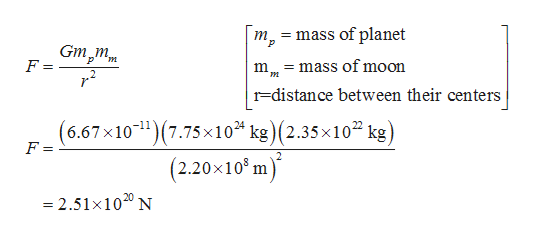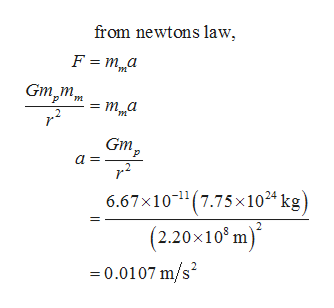# (A) Find the magnitude of the gravitational force (in N) between a planet with mass 7.75 ✕ 1024 kg and its moon, with mass 2.35 ✕ 1022 kg, if the average distance between their centers is 2.20 ✕ 108 m.  (B) What is the moon's acceleration (in m/s2) toward the planet? (Enter the magnitude.)m/s2  (C) What is the planet's acceleration (in m/s2) toward the moon? (Enter the magnitude.)m/s2

Question
7 views

(A) Find the magnitude of the gravitational force (in N) between a planet with mass 7.75 ✕ 1024 kg and its moon, with mass 2.35 ✕ 1022 kg, if the average distance between their centers is 2.20 ✕ 108 m.

(B) What is the moon's acceleration (in m/s2) toward the planet? (Enter the magnitude.)
m/s2

(C) What is the planet's acceleration (in m/s2) toward the moon? (Enter the magnitude.)
m/s
check_circle

Step 1

(a) The magnitude of gravitational force is given by,help_outlineImage Transcriptionclosem = mass of planet Gm m F = 2 = mass of moon r-distance between their centers (6.67x10(7.75x10 kg)(2.35x102 kg) (2.20x108 m' F = - 2.51x1020 N fullscreen
Step 2

(b) The Moons acceleration toward...help_outlineImage Transcriptionclosefrom newtons law F ma Gm,m = m a m т,а Gm а- 6.67x10 1(7.75 x 1024 kg) (2.20x10 m) -0.0107 m/s2 fullscreen

### Want to see the full answer?

See Solution

#### Want to see this answer and more?

Solutions are written by subject experts who are available 24/7. Questions are typically answered within 1 hour.*

See Solution
*Response times may vary by subject and question.
Tagged in

### Gravitation